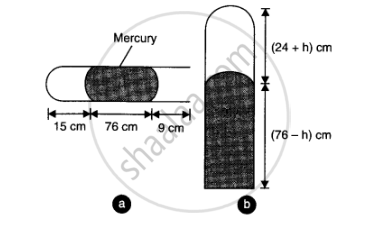CBSE (Science) Class 11CBSE
Share

# A Metre Long Narrow Bore Held Horizontally (And Closed at One End) Contains a 76 Cm Long Mercury Thread, Which Traps a 15 Cm Column of Air. What Happens If the Tube is Held Vertically with the Open End at the Bottom - CBSE (Science) Class 11 - Physics

ConceptSpecific Heat Capacities - Gases

#### Question

A metre long narrow bore held horizontally (and closed at one end) contains a 76 cm long mercury thread, which traps a 15 cm column of air. What happens if the tube is held vertically with the open end at the bottom?

#### Solution 1

When the tube is held horizontally, the mercury thread of length 76 cm traps a length of air = 15 cm. A length of 9 cm of the tube will be left at the open end. The pressure of air enclosed in tube will be atmospheric pressure. Let area of cross-section of the tube be 1 sq. cm.
.’. P= 76 cm and V= 15 cm3When the tube is held vertically, 15 cm air gets another 9 cm of air (filled in the right handside in the horizontal position) and let h cm of mercury flows out to balance the atmospheric pressure. Then the heights of air column and mercury column are (24 + h) cm and (76 – h) cm respectively.

The pressure of air = 76 - (76 - h) = h cm of mercury

:. V_2 = (24 + h) cm^3 and P_2 = h cm

if we assume that temperature remain constant then

P_1V_1 = P_2V_2 or 76 xx 15  = h xx (24 + h ) or h^2 + 24h - 1140 = 0

or h = (-24 +- sqrt((24)^2 + 4xx 1140))/2 = 23.8 cm or - 47.8 cm

Since h cannot be negative (because more mercury cannot flow into tube), there fore h = 23.8 cm

Thus in the verticle position of the tube , 23.8 cm of mercury flow out

#### Solution 2

Length of the narrow bore, L = 1 m = 100 cm

Length of the mercury thread, l = 76 cm

Length of the air column between mercury and the closed end, la = 15 cm

Since the bore is held vertically in air with the open end at the bottom, the mercury length that occupies the air space is: 100 – (76 + 15) = 9 cm

Hence, the total length of the air column = 15 + 9 = 24 cm

Let h cm of mercury flow out as a result of atmospheric pressure.

∴Length of the air column in the bore = 24 + h cm

And, length of the mercury column = 76 – h cm

Initial pressure, P1 = 76 cm of mercury

Initial volume, V1 = 15 cm3

Final pressure, P2 = 76 – (76 – h) = h cm of mercury

Final volume, V2 = (24 + h) cm3

Temperature remains constant throughout the process.

P1V1 = P2V2

76 × 15 = h (24 + h)

h2 + 24h – 1140 = 0

:. h = (-24+-sqrt((24)^2 + 4xx 1xx  1140))/(2xx1)

= 23.8 cm or –47.8 cm

Height cannot be negative. Hence, 23.8 cm of mercury will flow out from the bore and 52.2 cm of mercury will remain in it. The length of the air column will be 24 + 23.8 = 47.8 cm.

Is there an error in this question or solution?

#### Video TutorialsVIEW ALL 

Solution A Metre Long Narrow Bore Held Horizontally (And Closed at One End) Contains a 76 Cm Long Mercury Thread, Which Traps a 15 Cm Column of Air. What Happens If the Tube is Held Vertically with the Open End at the Bottom Concept: Specific Heat Capacities - Gases.
S